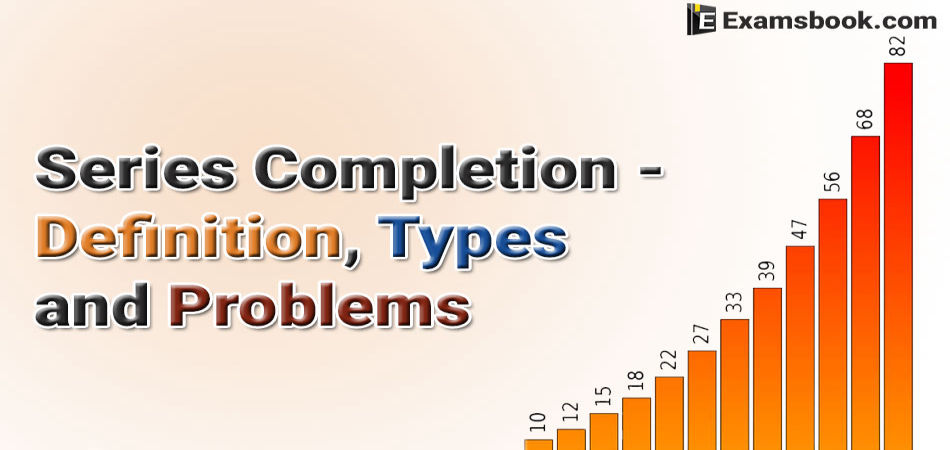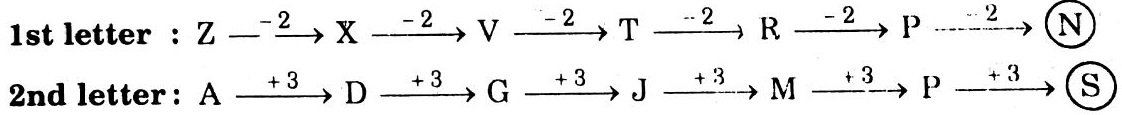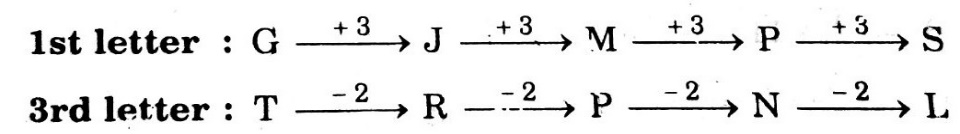• Save

# Series Completion - Definition, Types, Problems with Solutions

Last year 1.8K ViewsSeries completion questions are based on a special pattern of the series and you just need to identify the patterns of the given series. So, here I am providing information about series completion in details with its definition, types, problems with solutions and questions for your practice.

## Types of Series Completion in Detail:

### Definition of Series:

This chapter consists of questions in which series of number or alphabetical letters or combinations of both are given, which are generally called the terms of the series. These terms follow a certain pattern throughout the series. The candidate is required to study the given series, identify the pattern followed in the series and either complete the given series with the most suitable alternative or find the wrong ter1m in the series.

### Type 1: Number Series

Case I: Completing The Given Series by Finding the missing Terms(s)

Direction : Find the missing term in each of the following series:

Ex.1. 1, 6, 15, ? 45, 66, 91

(A) 25

(B) 26

(C) 27

(D) 28

Solution

Clearly the given sequence follows the pattern : +5, +9, +13, +17, +21, +25,………

Thus, 1+5 = 6, 6+9 = 15,……

So, missing term = 15+13 = 28. Hence, the answer is (d).

Ex.2. 2, 5, 9, 19, 37, ?

(A) 73

(B) 75

(C) 76

(D) 78

Solution

Clearly, we have : 2 × 2 + 1 = 5, 5 × 2 – 1 = 9, 9 × 2 + 1 = 19, 19 × 2 – 1 = 37,……

So, Missing term = 37 × 2 + 1 = 75. Hence, the answer is (b).

#### Pattern ofTriangular Series:

Sometimes, the difference between the consecutive terms of a series, again form a series. The difference between the consecutive terms of the new series so formed, again form a series. This pattern continues till we attain a uniform difference between the consecutive terms of series.

Ex.1. Find the missing term in the series : 3, 20, 63, 144, 275, ?

(A) 354

(B) 468

(C) 548

(D) 554

Solution

As discussed above, we may label the given series as series I and then form series II to IV as shown below:

Series I   :  3         20      63        144       275        ?

Series II  :      17       43         81       131       ?

Series III :            26        38        50         ?

Series IV :                    12       12

Clearly, the pattern in series III is + 12.

So, missing term in series III = 50 +12 = 62;

missing term in series II = 131 + 62 = 193;

missing term in series I = 275 + 193 = 468.

Thus, the missing term is 468. Hence, the answer is (b).

### Type 2: Alphabet Series:

In this type of questions, a series of single, pairs or groups of letters or combinations of letters and numerals is given. The terms of the series form a certain pattern as regards the position of the letters in the English alphabet. The candidate is required to decipher this pattern and accordingly find the missing term or the wrong term in the given series.

Example. Find the next two terms in the series : A, C, F, J, ?, ?

(A) L, P

(B) M, P

(C) O, U

(D) R, V

Solution

Clearly, the first, second, third,…………letters of the series are respectively moved two, three, four,………steps forward to obtain the successive terms of the series.

Thus, the fifth term in the series must be a letter which is five steps ahead of J i.e. O, while the sixth term must be a letter sixth term must be a letter six steps ahead of O i.e U.

Example.2. Which term comes next in the sequence: AC, FH, KM, PR, ?

(A) UW

(B) VW

(C) UX

(D) TV

(E) None of these

Solution

Clearly, the first and second letters of each term are moved five steps forward to obtain the corresponding letters of the next term.

Thus, the first letter of the missing term must be five steps ahead of P i.e U, while the second letter must be five steps ahead of R i.e. W. So, the missing term is UW. Hence, the answer is (a).

### Type 3: Alpha–Numeric Series:

This type of question is just a jumbled form of question of Type 1 and Type 2, which you have just read. Here, the terms of the given series are a combination of letters and numerals, study the following examples:

Ex.1. Find the next term in the alpha-numeric series:

Z1A, X2D, V6G, T21J, R88M, P445P, ?

(A) N2676S

(B) N2676T

(C) T2670N

(D) T2676N

Solution

Clearly, the patterns followed by the letters are as follows:The series formed by the numerals i.e 1,2,6,21, 88, 445,…….follows the pattern × 1 + 1, × 2 + 2, × 3 + 3, × 4 + 4, × 5 + 5, …….

So, numeral in the desired term = 445 × 6 + 6 = 2676.

Hence, desired term is N2676S.

Ex.2. Find the term which does not fit into the series Given below

G4T, J10R, M20P, P43N, S90L

(A) G4T

(B) J10R

(C) M20P

(D) P43N

(E) S901

Solution

The patterns followed by the letter are:The number series 4, 10, 20, 43, 90 should follow the pattern × 2 + 1, × 2 + 2, 2 +3, × 2 +4.

So, 10 is wrong and must be replaced by (4 × 2 + 1) i.e. 9.

Thus, the term J10R does not fit the given series. The correct term is J9R. Hence, the answer is (b).

If you have any concern about this topic, you can ask me anything in the comment section. For practice with solutions, go to the next page.